Courses

# Information Theory GATE Notes | EduRev

## GATE : Information Theory GATE Notes | EduRev

The document Information Theory GATE Notes | EduRev is a part of the GATE Course Communication System.
All you need of GATE at this link: GATE

Introduction:

Consider an arbitrary message denoted by xi. If the probability of the event that xi is selected for transmission, is given by

P (xi) = Pi

Then, the amount of the information associated with xis defined as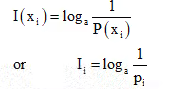Specifying the logarithmic base ‘a’ determines the unit of information. The standard convention of information theory takes a = 2 and the corresponding unit is the bit, i.e.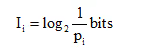The definition exhibits the following important properties:

a) If we are absolutely certain of the outcome of an event, even before it occurs, there is no information gained, i.e.

Ii = 0 for pi = 1

b) The occurrence of an event either provides some or no information, but never brings about a loss of information, i.e.

Ii> 0 for 0 < pi< 1

c) The less probable an event is, the more information we gain when it occurs.

Ij> I, for pj< pi

d) If two events xand xj are statistically independent, then

I(xixj) = I(xi) + I(xj)

Entropy:

Entropy of a source is defined as the average information associated with the source. Consider an information source that emits a set of symbols given by

X = {x1, x2, ………. xn)

If each symbol xi occurs with probability pi and conveys the information Ii, then the average information per symbol is obtained as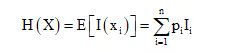This is called the source entropy. Again, substituting equation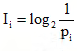into above expression, we get the more generalized form of source entropy as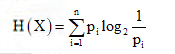Properties of Entropy:

Following are some important properties of source entropy.

a) In a set of symbol X, if the probability pi = 1 for some i, and the remaining probabilities in the set are all zero; then the entropy of the source is zero, i.e

H(X) = 0

b) If all the n symbols emitted from a source are equiprobable, then the entropy of the source is

H(X) = log2n

c) From above two results, we can easily conclude that the source entropy is bounded as

0 < H(X) < log2n

Information Rate:

The information rate (source rate) is defined as the average number of bits of information per second generated from the source. Thus, the information rate for a source having entropy H is given by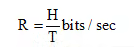where T is the time required to send a message. If the message source generates messages at the rate of r messages per second, then we have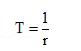Substituting it in above equation, we get the more generalized expression for the information rate of the source as

R = rH bits / sec

Source Coding:

An important problem in communication is the efficient representation of data generated by a discrete source. The process by which this representation is accomplished is called source encoding. Our primary interest is in the development of an efficient source encoder that satisfies two functional requirements:

a) The code words produced by the encoder are in binary from.

b) The source code is uniquely decodable, so that the original source sequence can be reconstructed perfectly from the encoded binary sequence.

Average code – word length:

Assume that the source has a set of n different symbols, and that the ith symbol xi occurs with probability

P(xi) = pi where i = 1,2, ……… n

Let the binary code – word assigned to symbol xi by the encoder have length Ii measured in bits. Then, the average code – word length is defined as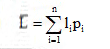Source Coding theorem:

According to source encoding theorem, the minimum average code – word length for any distortion less source encoding scheme is defined as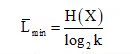Where H(X) is the entropy of the source, and k is the number of symbols in encoding alphabet. Thus, for the binary alphabet (k = 2), we get the minimum average code – word length as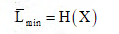Coding Efficiency:

The coding efficiency of a source encoder is defined as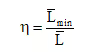Where is the minimum possible value of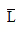. Substituting the value of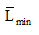in above expression, we get the more generalized form of coding efficiency as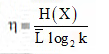Where H(X) is the entropy of the source, k is the number of symbols in encoding alphabet, andis the average code – word length. Thus, for the binary alphabet (k = 2), we get the coding efficiency as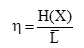Classification of Codes:

Shannon-Fano Coding:

An efficient code can be obtained by the following simple procedure, known as

Shannon- Fano algorithm.

The Shannon – Fano coding is very easy to apply and usually yields source codes having reasonably high efficiency.

Methodology: Shannon – Fano encoding algorithm:

Following are the steps involved in Shannon – Fano coding of a source symbol:

Step 1: The source symbols are first ranked in order of decreasing probability.

Step 2: The set is then partitioned into two sets that are as close to equiprobable as possible

Step 3: 0’s are assigned to the upper set and 1’s to the lower set.

Step 4: The above process is continued, each time partitioning the sets with as nearly equal probabilities as possible, until further partitioning is not possible.

Or we can represent this coding as:

1. List the source symbols in order of decreasing probability.
2. Partition the set into two sets that are as close to equiprobable as possible, and assign 0 to the upper set and 1 to the lower set.
3. Continue this process, each time partitioning the sets with as nearly equal probabilities as possible until further partitioning is not possible

It can be understood by the example given below-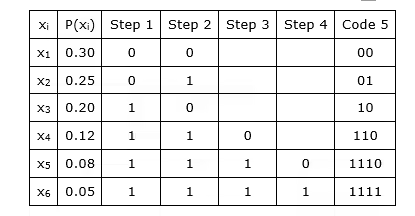Huffman coding:

Basically, Huffman coding is used to assign to each symbol of an alphabet a sequence of bits roughly equal in length to the amount of information conveyed by the symbol in question.

Methodology: Huffman encoding algorithm:

Following are the steps involved in Huffman encoding coding of a source symbol:

Step 1: The source symbols are listed in order of decreasing probability.

Step 2: The two source symbols of lowest probability are assigned a 0 an a 1.

Step 3: These two source symbols are regarded as being combined into a new source symbol with probability equal to the sum of the two original probabilities. (the list source symbols, and therefore source statistics, is thereby reduced in size by one.)

Step 4: The probability of the new symbol is placed in the list in accordance with its value.

Step 5: The above procedure is repeated until we are left with a final list of source statistics (symbols) of only two for which a 0 and a 1 are assigned.

Step 6: The code for each (original) source symbol is found by working backward and tracing the sequence of 0s and 1s assigned to that symbol as well as its successors.

Or we can understand Huffman coding as:

In general, Huffman encoding results in an optimum code. Thus, it is the code that has the highest efficiency . The Huffman encoding procedure is as follows:

1. List the source symbols in order of decreasing probability.
2. Combine the probabilities of the two symbols having the lowest probabilities, and reorder the resultant probabilities; this step is called reduction 1. The same procedure is repeated until there are two ordered probabilities remaining.
3. Start encoding with the last reduction, which consists of exactly two ordered probabilities. Assign 0 as the first digit in the code words for all the source symbols associated with the first probability; assign 1 to the second probability.
4. Now go back and assign 0 and 1 to the second digit for the two probabilities that were combined in the previous reduction step, retaining all assignments made in Step 3.
5. Keep regressing this way until the first column is reached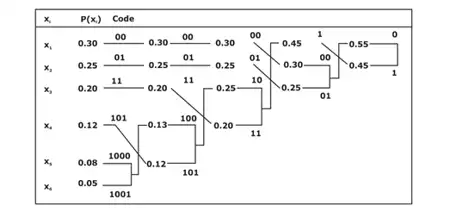Discrete Channel Models:

Discrete memoryless channels are completely specified by the set of conditional probabilities that relate the probability of each output state to the input probabilities.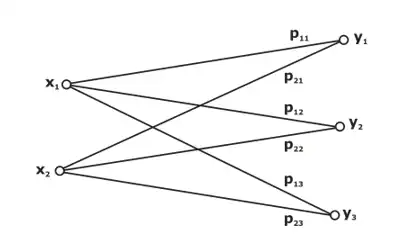Channel Matrix: (Channel Transition probability):

In the discrete channel models, each possible input – to – output path is indicated along with a conditional probability pij, which is concise notation for P(yj|xi). Thus pij is the probability of obtaining output yj given that the input is xand is called a channel transition probability. The discrete channel shown in above figure, is specified by the matrix of transition probabilities as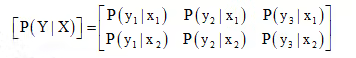If the probabilities of channel input X and output Y are represented by the row matrix as

[P(X)] = [p(x1)p(x2)]

and

[P(Y)] = [p(y1)p(y2)p(y3)]

Then, the relation between the input and output probabilities are given by

[P(Y)] = [P(X)][P(Y|X)]

In general, we can understand it as:

A channel is completely specified by the complete set of transition probabilities.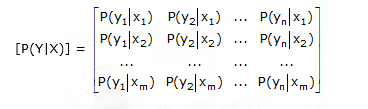If P(X) is represented as a diagonal matrix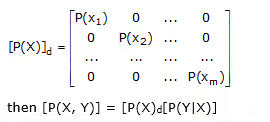where the (i, j) element of matrix [P(X, Y)] has the form P(xi, yj). The matrix [P(X, Y)] is known as the joint probability matrix, and the element P(xi,yj) is the joint probability of transmitting xi and receiving yj.

Entropy functions for Discrete Memoryless Channel:

Consider a discrete memoryless channel with the input probabilities P(xi), the output probabilities P(yj), the transition probabilities P(yj | xi), and the joint probabilities P(xi, yj). If the channel has n inputs and m outputs, then we can define several entropy functions for input and output as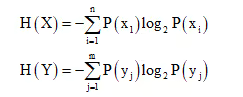a) Joint Entropy

The joint entropy of the system is obtained as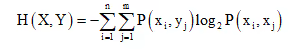b) Conditional Entropy

The several conditional entropy functions for the discrete memoryless channel is defined as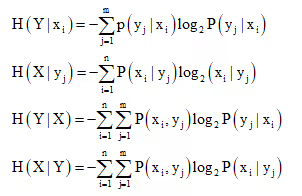Mutual Information:

The mutual information I(X; Y) of a channel is defined by

I(X; Y) = H(X) – H(X|Y) b/symbol

Since H(X) represents the uncertainty about the channel input before the channel output is observed and H(X|Y) represents the uncertainty about the channel input after the channel output is observed, the mutual information I(X; Y) represents the uncertainty about the channel input that is resolved by observing the channel output.

Also, we can define the mutual information as

I (X; Y) = H(Y) – H(Y | X)

Channel Capacity:

The channel capacity is defined as the maximum of mutual information, i.e.

C = max {I(X;Y)}

Substituting above equation, we get the channel capacity as

C = max {H(X) – H(X |Y)}

This result can be more generalized for the Gaussian channel. The information capacity of a continuous channel of bandwidth B hertz is defined as

C = Blog2 (1 + S/N)

where S/N is the signal to noise ratio. This relationship is known as the Hartley – Shannon law that sets an upper limit on the performance of a communication system.

Channel Efficiency:

The channel efficiency is defined as the ratio of actual transformation to the maximum transformation, i.e.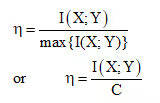Special Channels:

Lossless Channel:

A channel described by a channel matrix with only one nonzero element in each column is called a lossless channel. An example of a lossless channel is shown in Figure below, and the corresponding channel matrix is shown below equation.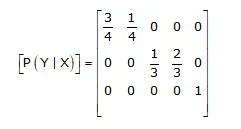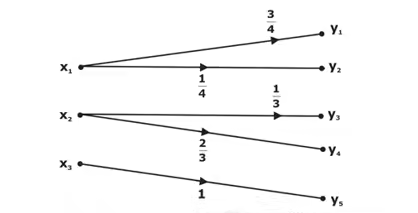Deterministic Channel:

A channel described by a channel matrix with only one nonzero element in each row is called a deterministic channel. An example of a deterministic channel is shown in below figure, and the corresponding channel matrix is shown in below equation.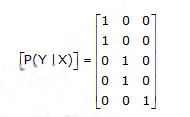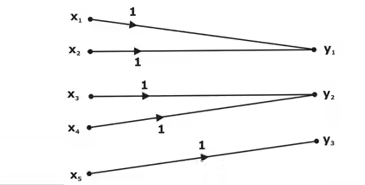Noiseless Channel:

A channel is called noiseless if it is both lossless and deterministic. A noiseless channel is shown in below figure. The channel matrix has only one element in each row and in each column, and this element is unity. Note that the input and output alphabets are of the same size; that is, m = n for the noiseless channel.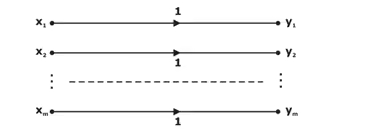Binary Symmetric Channel:

The binary symmetric channel (BSC) is defined by the channel diagram shown in below figure, and its channel matrix is given by: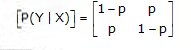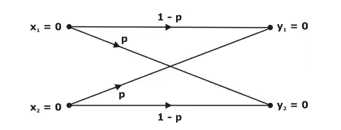Offer running on EduRev: Apply code STAYHOME200 to get INR 200 off on our premium plan EduRev Infinity!

10 docs|12 tests

,

,

,

,

,

,

,

,

,

,

,

,

,

,

,

,

,

,

,

,

,

;